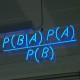## an unbiased estimator of the Hellinger distance?

Here is a question I posted on Stack Exchange a while ago:

In a setting where one observes X1,…,Xn distributed from a distribution with (unknown) density f, I wonder if there is an unbiased estimator (based on the Xi‘s) of the Hellinger distance to another distribution with known density f0, namely$\mathfrak{H}(f,f_0)=\left\{1-\int\sqrt{f_0(x)/(x)}\text{d}x\right\}^{1/2}$
Now, Paulo has posted an answer that is rather interesting, if formally “off the point”. There exists a natural unbiased estimator of if not of H, based on the original sample and using the alternative representation$\mathfrak{H}^2(f,f_0)=1-\mathbb{E}_f[\sqrt{f_0(X)/f(X)}]$

for the Hellinger distance. In addition, this estimator is guaranteed to enjoy a finite variance since$\mathbb{E}_f[\sqrt{f_0(X)/f(X)}^2]=1\,.$

Considering this question again, I am now fairly convinced there cannot be an unbiased estimator of H, as it behaves like a standard deviation for which there usually is no unbiased estimator!

### 3 Responses to “an unbiased estimator of the Hellinger distance?”

1.Estimador não-viesado para o desvio-padrão | Blog Pra falar de coisas Says:

[…] Acabei de descobrir que não existe estimador não-viesado para o desvio-padrão. Sempre aprendi que havia um estimador não-viesado para a variância. Que os livros (e os professores)  silenciassem sobre um estimador não-viesado para o desvio-padrão nunca me chamou a atenção. Afinal, parecia natural que haveria um estimador não-viesado para o desvio padrão: a raiz quadrada da variância amostral. Porém, isso não funciona. Felizmente sou Bayesiano e não me preocupo com o viés. […]

•xi'an Says:

Thanks. This is indeed of my favourite arguments against unbiasedness as a relevant criterion. There was another similar question on Stack Exchange about unbiased Bayes estimators (they do no exist!).

2.Paulo. Says:

You intuition is probably right. We should have a proof of that.

This site uses Akismet to reduce spam. Learn how your comment data is processed.